# 图的学习总结Nick1 什么是图？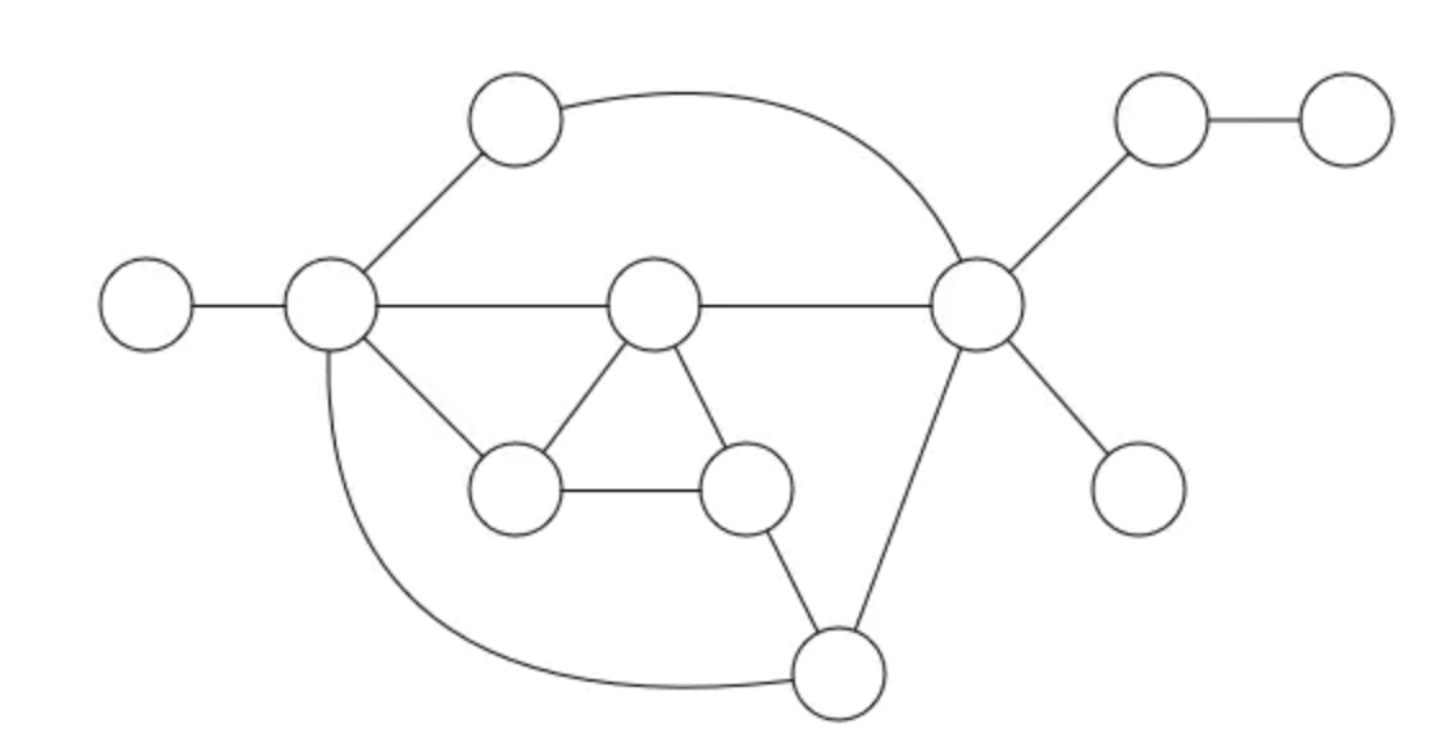2 什么是有向图和无向图？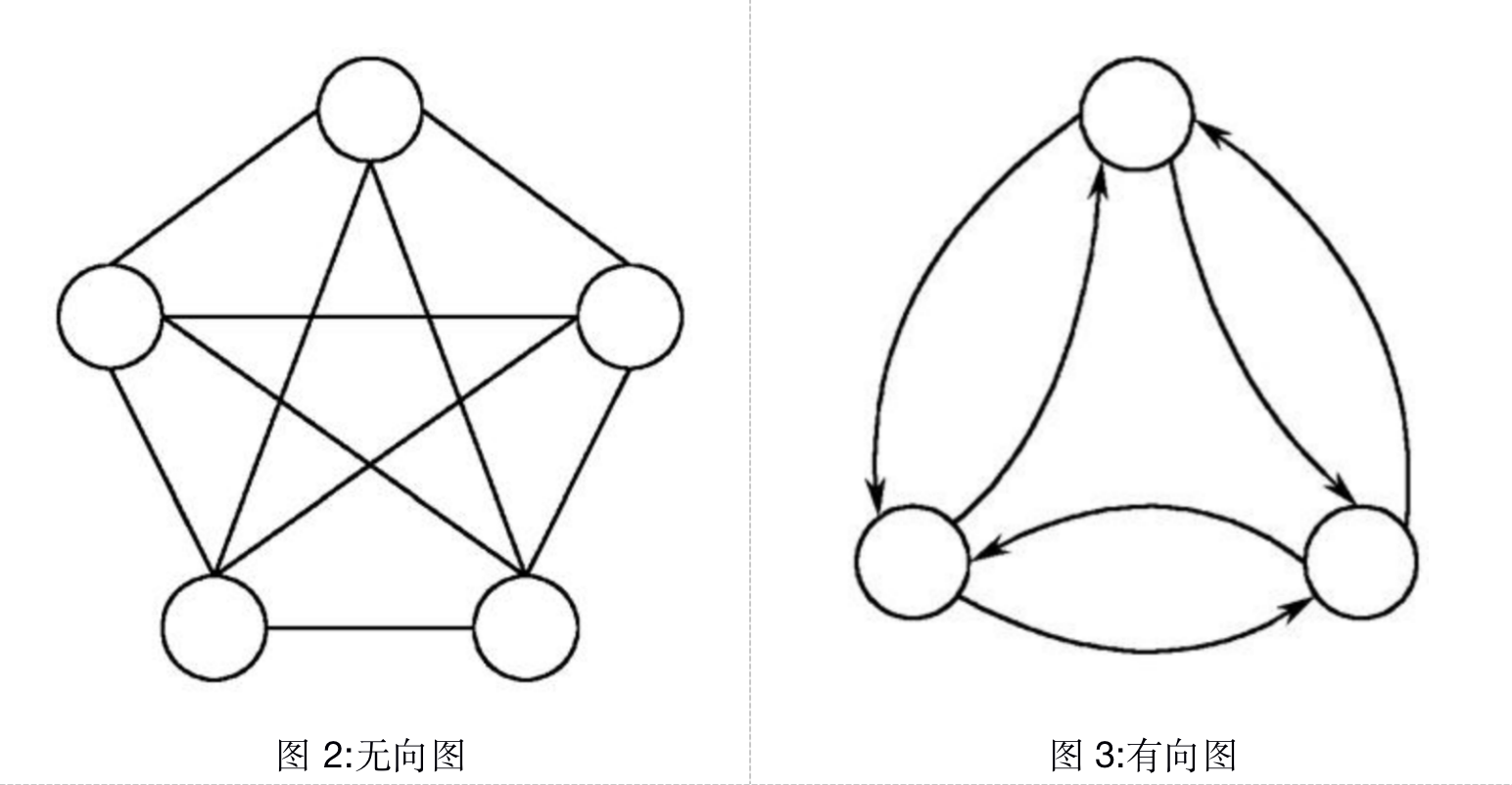3 什么是度以及什么是入度和出度？

4 什么是带权图？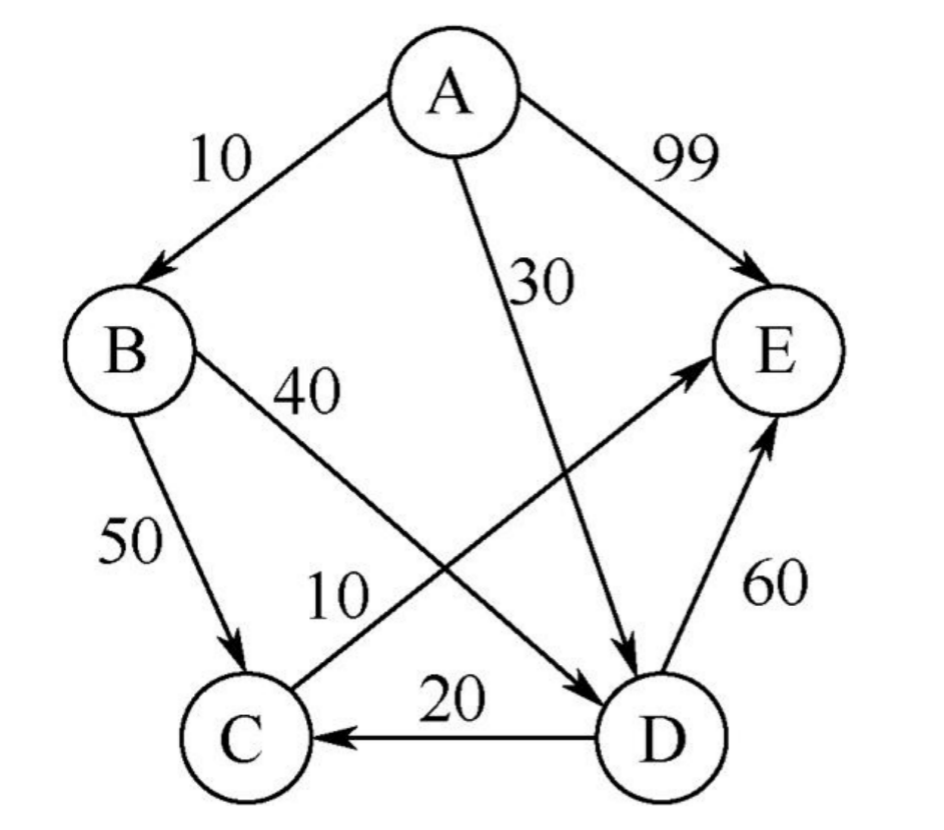5 如何存储一个图？

5.1 邻接矩阵存储方法

ü 存储方式简单、直接，因为基于数组，所以在获取两个顶点的关系时，就非常高效。

ü 方便计算。这是因为，用邻接矩阵的方式存储图，可以将很多图的运算转换成矩阵之间的运算。

ü 浪费存储空间，如果我们存储的是稀疏图（Sparse Matrix），也就是说，顶 点很多，但每个顶点的边并不多，那邻接矩阵的存储方法就更加浪费空间了。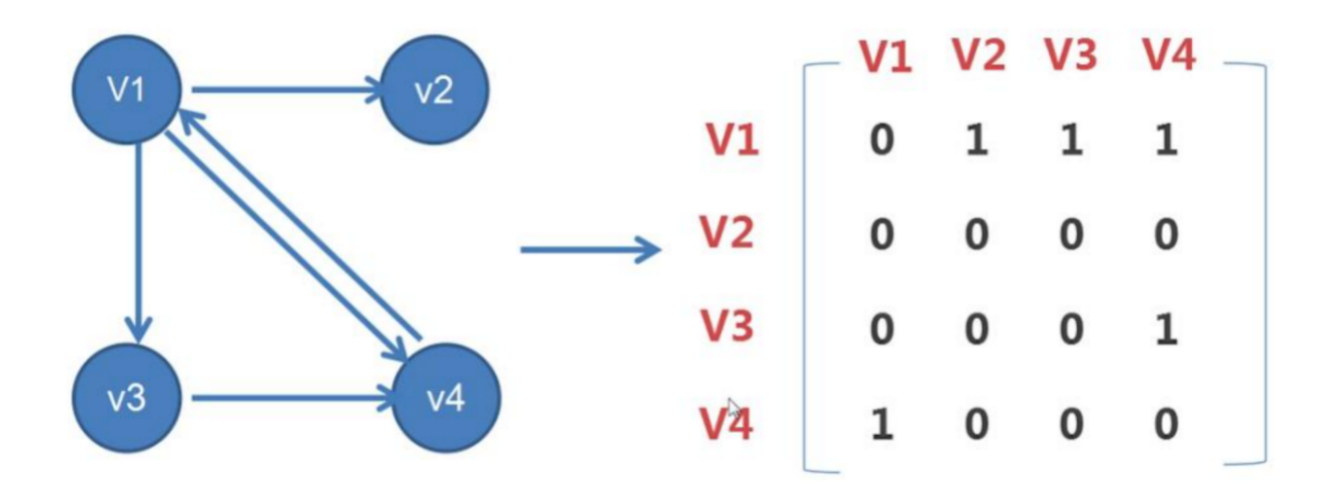5.2 邻接表存储方法

ü 节省存储空间

ü 使用起来比较耗时

ü 将邻接表中的链表改成平衡二叉查找树。实际开发中，我们可以选择用红黑树。这样，我们就可以更加快速地查找两个顶点之间是否存在边了

ü 将链表改成有序动态数组，可以通过二分查找的方法来快速定位两个顶点之间否是存在边。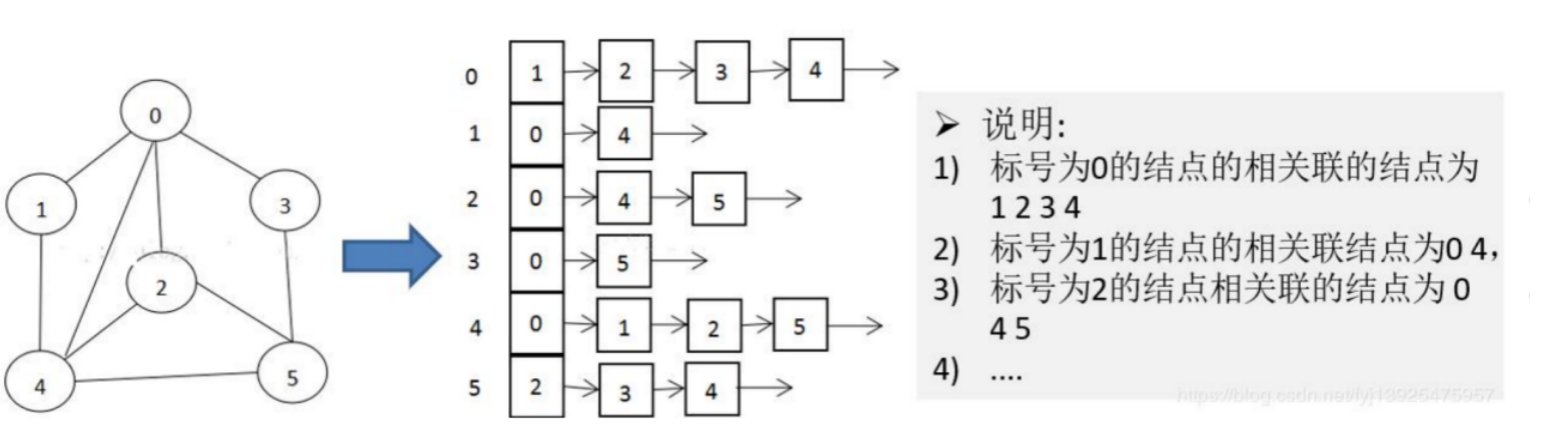6 图的应用和实现？

6.1 图的应用-什么是 BFS、DFS？

DFS：深度优先搜索（Depth-First-Search），简称 DFS。最直观的例子就是“走迷宫”。

6.2 图的代码实现

BFS 的代码实现

public void bfs(int s, int t) {  if (s == t) return;  boolean[] visited = new boolean[v];  visited[s]=true;  Queue<Integer> queue = new LinkedList<>();  queue.add(s);  int[] prev = new int[v];  for (int i = 0; i < v; ++i) {    prev[i] = -1;  }  while (queue.size() != 0) {    int w = queue.poll();   for (int i = 0; i < adj[w].size(); ++i) {      int q = adj[w].get(i);      if (!visited[q]) {        prev[q] = w;        if (q == t) {          print(prev, s, t);          return;        }        visited[q] = true;        queue.add(q);      }    }  }}private void print(int[] prev, int s, int t) { // 递归打印s->t的路径  if (prev[t] != -1 && t != s) {    print(prev, s, prev[t]);  }  System.out.print(t + " ");}

DFS 的代码实现

boolean found = false; // 全局变量或者类成员变量public void dfs(int s, int t) {  found = false;  boolean[] visited = new boolean[v];  int[] prev = new int[v];  for (int i = 0; i < v; ++i) {    prev[i] = -1;  }  recurDfs(s, t, visited, prev);  print(prev, s, t);}private void recurDfs(int w, int t, boolean[] visited, int[] prev) {  if (found == true) return;  visited[w] = true;  if (w == t) {    found = true;    return;  }  for (int i = 0; i < adj[w].size(); ++i) {    int q = adj[w].get(i);    if (!visited[q]) {      prev[q] = w;      recurDfs(q, t, visited, prev);    }  }}## 评论